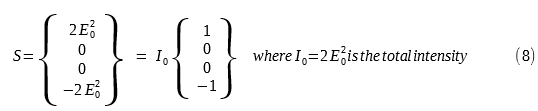# Stokes Vector

### The Definition of Stokes VectorWe can arrange the four Stokes parameters into a column matrix as: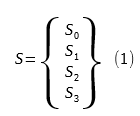Mathematically, it is not a vector, but through custom it is called the Stokes vector.

From the Stokes parameters definition (12), (13), (14), (15) above, the Stokes vector for elliptically polarized light is written as: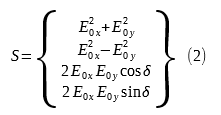(2) is also called the Stokes vector for a plane wave.

### Stokes Vectors for Linearly and Circularly Polarized Light

Just how we found the Stokes parameters in this article, we can find the Stokes vectors for linearly and circularly polarized light.

#### 1. Linear Horizontally Polarized Light (LHP)

In this case, E0y = 0, from (2) we can get the Stokes vector as:#### 2. Linear Vertically Polarized Light (LVP)

In this case, E0x = 0, from (2) we can get the Stokes vector as:#### 3. Linear +45° Polarized Light (L +45)

In this case, we have

• E0x =  E0y = E0
• δ = δy - δx = 0

The Stokes vector is: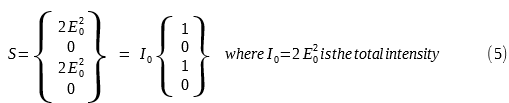#### 4. Linear -45° Polarized Light (L -45)

In this case, we have

• E0x =  E0y = E0
• δ = δy - δx = 180°

The Stokes vector is: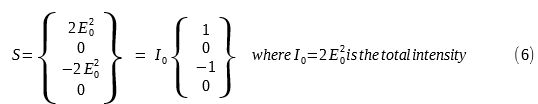#### 5. Right Circularly Polarized Light (RCP)

In this case, we have

• E0x =  E0y = E0
• δ = δy - δx = 90°

And the Stokes vector is: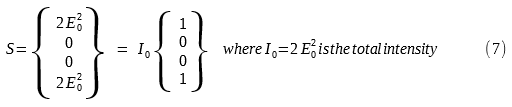#### 6. Left Circularly Polarized Light (LCP)

In this case, we have

• E0x =  E0y = E0
• δ = δy - δx = - 90°

The Stokes vector is: